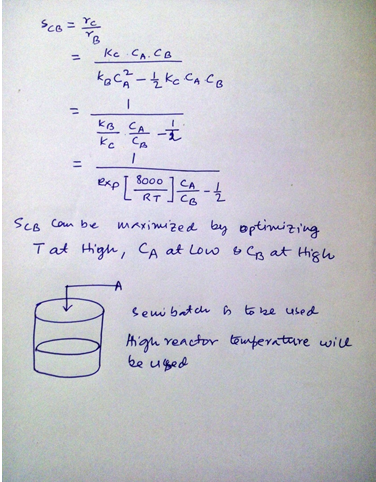# Question & Answer: The following elementary liquid phase reactions are to be carried out In the reactors A – rightarrow B…

The following elementary liquid phase reactions are to be carried out In the reactors A – rightarrow B r_B = k_B C_A^2 A + B – rightarrow -2C r_C = k_C C_A C_B What is the instantaneous selectivity of C to B? Which reactor or combination of reactors and at what temperatures would you use for the following reaction system? C is the desired product. Data C_A 0 = 4 mol/dm^3 k_B = 1 dm^3/mol middot min at 300K with E = 4000cal/mol k_C = 1 dm^3/mol middot min at 300K with E = 12000cal/mol